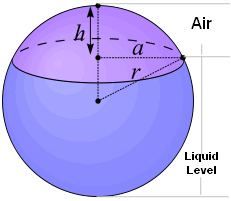## Partially Filled Sphere Calculator

>>
>>
The input number unit is? or

Significant Figures >>>

For ease of calculating, we chose 1) liquid level and 2) tank diameter as the data items required for input.

If you only know cap height and diameter, just remember this simple relationship:
Liquid Level = Diameter - Cap Height.If you need a calculator that will accept other input units, then click here.

The total volume of a partially-filled spherical tank equals total sphere volume minus spherical cap volume.